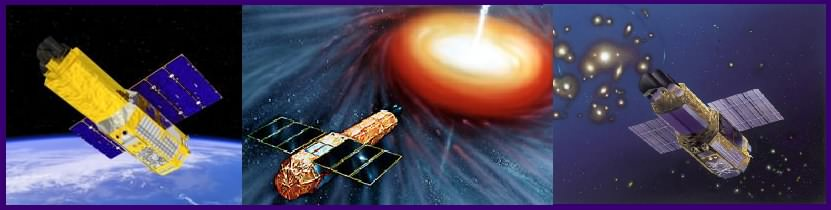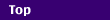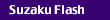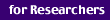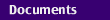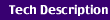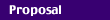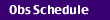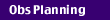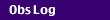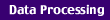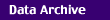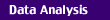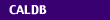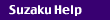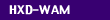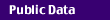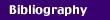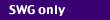Top > Suzaku > Observation Planning > XIS telemetry limit

## XIS telemetry limit

 2006/05/25 Tadayasu Dotani, Hideyuki Mori 2007/01/09 Tadayasu Dotani, Hideyuki Mori, Uchiyama Hideki

### 1. Upper limits of count rates

Upper limits of count rates (source + background), which are determined only by the telemetry allocation for the XIS, are summarized in the following table. Values in parentheses represent maximum count rates when the 3 sigma statistical fluctuation is taken into consideration.
(revised on 2006/12/19 after the XIS2 trouble)

 Data rate/Edit mode 5x5 3x3 2x2 timing Super High 138(126) 291(274) 614(589) 1382(1344) High 138(126) 291(274) 614(589) 1382(1344) Medium (weekday) 58(50) 121(110) 256(240) 576(551) Medium (weekend) 24(19) 51(44) 107(96) 240(224) Low (weekday) 14(11) 30(25) 64(56) 144(132)
in units of counts/sec/sensor

#### Note:

(1) These count rates represent only approximate values, since the telemetry consumption depends on an efficiency of data compression.

(2) We assume a distribution of the telemetry allocation among the 4 sensors as XIS0:XIS1:XIS2:XIS3 = 3:3:1:3, which was changed from the even distribution on Dec. 20, 2006.

#### Supplementary explanation:

(1) Backgrounds

The Non-X-ray Background rates are normally 10 cts/s for the FI CCDs and 20 cts/s for the BI CCD, when the event threshold for the FI CCD is set to 100 adu. These values represent the 90% upper limits of variations in background count rates.

(2) Current telemetry distribution among XRS, XIS, and HXD (revised on 2006/03/23)

 Data rate Weekday Weekend SH 10/144/66 --- H 10/144/66 --- M 1/60/40 1/25/40 L 1/15/10 1/1/10
in units of kbps

(3) Compressed data size per event

 Edit mode Minimum Maximum Average 5x5 28 52 40 3x3 15 23 19 2x2 8 11 9 Timing 4 4 4
in units of byte/cts

We show an example in which the upper limit of count rate in the Data rate "High", 5x5 mode is calculated.

Since the telemetry allocation for the XIS in the Data rate "High" is 144 kbps = 144 * 1024 / 8 = 18432 byte/sec (See the table of the current telemetry distribution), the count rate of 18432 (byte/sec) / 40 (byte/cts) * 3 / (3 + 3 + 1 + 3) (sensor) = 138 cts/s is the maximum that does not cause the telemetry saturation for the XIS0, 1, and 3.

(4) Data Recorder consumption

If the count rates per sensor are assumed to be A cts/s (FI CCDs) and B cts/s (BI CCD), you can estimate the required telemetry as follows:
 5x5: 2.976 + 0.313*(7/3*A + B) kbps 3x3: 2.976 + 0.148*(7/3*A + B) kbps
The constant (i.e., 2.976 kbps) corresponds to the telemetry assigned for the header of each frame.

The scaling factors are given by:
 40 (byte/cts) * 8 (bit) / 1024 = 0.313 (kbit/cts) 19 (byte/cts) * 8 (bit) / 1024 = 0.148 (kbit/cts)

(5) Pile-up

In the Normal mode, the upper limits of source count rates are usually determined by the photon pile-up rather than the telemetry limits.

### 2. Upper limits of source count rates (examples of the estimation)

(1) Upper limit of source count rate in the weekend observation (Data rate "Medium"):

In the Data rate "Medium", the upper limits of background- subtracted count rates are 34 cts/s (= 44 - 10 cts/s) for FI CCDs and 24 cts/s (= 44 - 20 cts/s) for BI CCD in the 3x3 mode.

(2) Upper limit of source count rate in the weekday observation (Data rate "Low"):

Same as the Data rate "Medium", the upper limits of background- subtracted count rates are 15 cts/s (= 25 - 10 cts/s) for FI CCDs and 5 cts/s (= 25 - 20 cts/s) BI CCD in the Data rate "Low", 3x3 mode.

ISAS/JAXA Department of High Energy Astrophysics